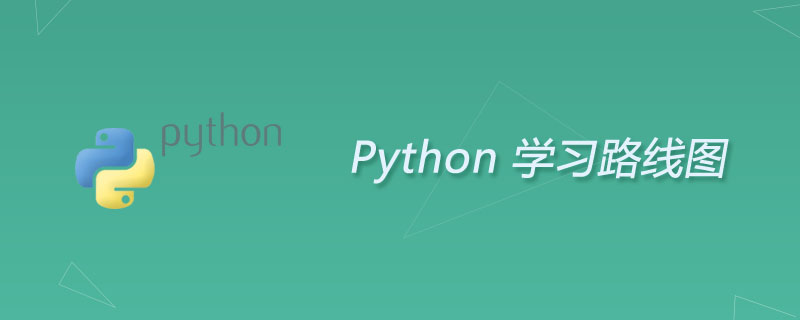python实现多进程实现多进程

基本使用

Semaphore（信号量）、Pipe（管道）、Lock（锁）、Pool（进程池）等。

import multiprocessing
import time

now = lambda: time.time()

def work(index):
print(index)
time.sleep(index)

def main():
start_dt = now()
for i in range(5):
p = multiprocessing.Process(target=work, args=(i,))
p.start()

print(f"Time: {now() - start_dt}")

if __name__ == "__main__":
main()

多进程基本信息的获取

import multiprocessingimport timenow = lambda: time.time()def work(i, index):    print(f'{i}进程启动')    time.sleep(index)    print(f'{i}进程结束')def main():    start_dt = now()    for i in range(5):        p = multiprocessing.Process(target=work, args=(i, 5))        p.start()    # 查看本机的 cpu 数量    print(f"CPU Numbers: {multiprocessing.cpu_count()}")    # 查看全部活跃子进程的名称以及pid    for p in multiprocessing.active_children():        print(p.name, p.pid)    print(f"Time End: {now() - start_dt}")if __name__ == "__main__":    main()

继承 Process 类

import multiprocessing
import time

class MyProcess(multiprocessing.Process):
def __init__(self, loop):
super(MyProcess, self).__init__()
self.loop = loop

def run(self):
for count in range(self.loop):
time.sleep(1)
print(f"Pid: {self.pid}, Name: {self.name}")

def main():
for i in range(2, 5):
p = MyProcess(i)
p.start()

if __name__ == "__main__":
main()

守护进程

import multiprocessing
import time

class MyProcess(multiprocessing.Process):
def __init__(self, loop):
super(MyProcess, self).__init__()
self.loop = loop

def run(self):
for count in range(self.loop):
time.sleep(1)
print(f"Loop: {self.loop}, Pid: {self.pid}, Loop Count: {count}")

def main():
for i in range(2, 5):
p = MyProcess(i)
p.daemon = True
p.start()

if __name__ == "__main__":
main()

# 主进程没有做任何事情 直接输入后结束 同时也终止了子进程的运行。
print("Main End.")

进程等待

import multiprocessing
import time

class MyProcess(multiprocessing.Process):
def __init__(self, loop):
super(MyProcess, self).__init__()
self.loop = loop

def run(self):
for count in range(self.loop):
time.sleep(1)
print(f"Loop: {self.loop}, Pid: {self.pid}, Loop Count: {count}")

def main():
processes = []
for i in range(2, 5):
p = MyProcess(i)
p.daemon = True
p.start()
processes.append(p)

for p in processes:
p.join()

if __name__ == "__main__":
main()

# 主进程没有做任何事情 直接输入后结束 同时也终止了子进程的运行。
print("Main End.")

进程等待最大时间

import multiprocessing
import time

class MyProcess(multiprocessing.Process):
def __init__(self, loop):
super(MyProcess, self).__init__()
self.loop = loop

def run(self):
for count in range(self.loop):
time.sleep(1)
print(f"Loop: {self.loop}, Pid: {self.pid}, Loop Count: {count}")

def main():
processes = []
for i in range(2, 5):
p = MyProcess(i)
p.daemon = True
p.start()
processes.append(p)

for p in processes:
# 主进程最多等待改进程 1 s
p.join(1)

if __name__ == "__main__":
main()

# 主进程没有做任何事情 直接输入后结束 同时也终止了子进程的运行。
print("Main End.")

终止进程

import multiprocessing
import time

print("1")
time.sleep(5)
print("2")

if __name__ == "__main__":
# 使用 is_alive 判断当前进程进程是否在运行
print(f"First: {p}, {p.is_alive()}")

p.start()
print(f"During: {p}, {p.is_alive()}")

p.terminate()
# 即使此时已经调用了 terminate 进程的状态还是 True, 即运行状态
# 在调用 join 之后才变成了终止状态
print(f"After: {p}, {p.is_alive()}")

p.join()
print(f"Joined: {p}, {p.is_alive()}")

进程互斥锁

import multiprocessing
import time

class MyProcess(multiprocessing.Process):
def __init__(self, loop, lock: multiprocessing.Lock):
super(MyProcess, self).__init__()
self.loop = loop
self.lock = lock

def run(self):
for count in range(self.loop):
time.sleep(0.1)
# self.lock.acquire()
print(f"Pid: {self.pid}, LoopCount: {count}")
# self.lock.release()

def main():
lock = multiprocessing.Lock()
for i in range(10, 15):
p = MyProcess(i, lock)
p.start()

if __name__ == "__main__":
main()

信号量

import multiprocessing
import time

'''
Semaphore管理一个内置的计数器，

'''

buffer = multiprocessing.Queue(10)
empty = multiprocessing.Semaphore(2)   # 缓冲区闲适区空余数
full = multiprocessing.Semaphore(0)    # 缓冲区占用区占用数
lock = multiprocessing.Lock()

class Consumer(multiprocessing.Process):
def run(self):
global buffer, empty, full, lock
while True:
full.acquire()
lock.acquire()
buffer.get()
print("Consumer pop an element.")
time.sleep(1)
lock.release()
empty.release()

class Producer(multiprocessing.Process):
def __init__(self, name):
super(Producer, self).__init__()
self.name = name

def run(self):
global buffer, empty, full, lock
# 生产者 Producer 使用 acquire 方法来占用一个缓冲区位置，缓冲区空闲区大小减 1，接下来进行加锁，对缓冲区进行操作，
# 然后释放锁，最后让代表占用的缓冲区位置数量加 1，消费者则相反。
# 通过 Semaphore 我们很好地控制了进程对资源的并发访问数量。
while True:
empty.acquire()
lock.acquire()
buffer.put(1)
print(f"{self.name} Producer put an element.")
time.sleep(1)
lock.release()
full.release()

def main():
lst = []
for i in range(3):
p = Producer(str(i))
p.daemon = True
p.start()
lst.append(p)

c = Consumer()
c.daemon = True
c.start()
c.join()

for p in lst:
p.join()

print("Main End.")

if __name__ == "__main__":
main()

管道

import multiprocessing

class Consumer(multiprocessing.Process):
def __init__(self, pipe):
super(Consumer, self).__init__()
self.pipe = pipe

def run(self):
self.pipe.send("Consumer Words.")
print(f"Consumer Recv: {self.pipe.recv()}")

class Producer(multiprocessing.Process):
def __init__(self, pipe):
super(Producer, self).__init__()
self.pipe = pipe

def run(self):
self.pipe.send("Producer Words.")
print(f"Producer Recv: {self.pipe.recv()}")

def main():
# 声明了一个默认为双向的管道，然后将管道的两端分别传给两个进程。
# 管道 Pipe 就像进程之间搭建的桥梁，利用它我们就可以很方便地实现进程间通信了。
pipe = multiprocessing.Pipe()
c = Consumer(pipe)
p = Producer(pipe)
c.daemon = True
p.daemon = True
c.start()
p.start()
c.join()
p.join()
print("Main Process Ended.")

if __name__ == "__main__":
main()

进程池

Pool可以提供指定数量的进程，供用户调用，当有新的请求提交到pool中时，如果池还没有满，就会创建一个新的进程用来执行该请求；

import multiprocessing
import time

def function(index):
print(f"{index} start")
time.sleep(3)
print(f"{index} end")

def main():
# 声明了一个大小为 3 的进程池，通过 processes 参数来指定，如果不指定，那么会自动根据处理器内核来分配进程数。
pool = multiprocessing.Pool(processes=3)
for i in range(4):
# 使用 apply_async 方法将进程添加进去，args 可以用来传递参数。
pool.apply_async(function, args=(i, ))

print("Main start.")
# 关闭进程池 使之不再接受新任务
pool.close()
pool.join()
print("Main end.")

if __name__ == "__main__":
main()

import multiprocessing
import requests

def scrape(url):
try:
ret = requests.get(url).text
print(ret[:10])
print()
except:
print("Get Error")

def main():
pool = multiprocessing.Pool(processes=3)
urls = [
'http://data.eastmoney.com/hsgt/index.html',
'http://data.eastmoney.com/hsgtcg/gzcglist.html',
'https://www.runoob.com/mysql/mysql-alter.html',

]
pool.map(scrape, urls)
pool.close()
# pool.join()
print("Main End.")

if __name__ == "__main__":
main()

Python开发开发项目